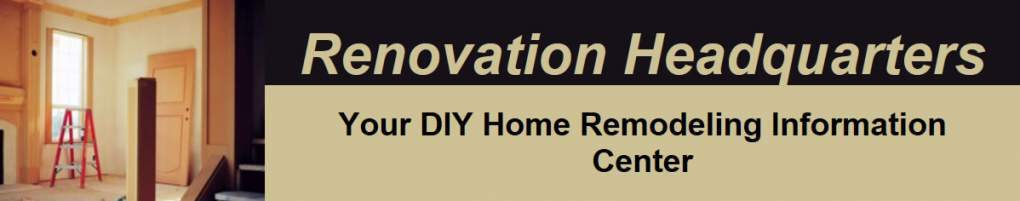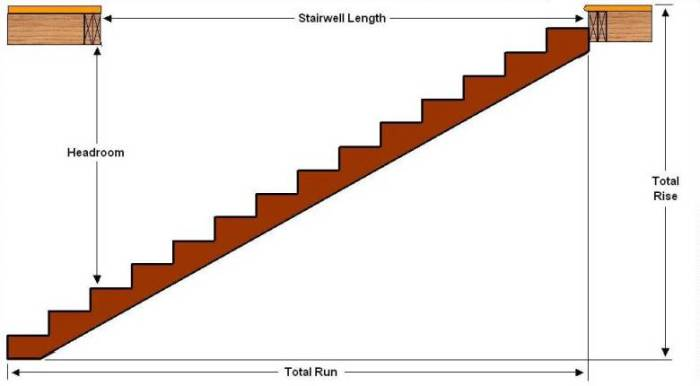# How To Build Stairs - Part 1b

### Letters in Orange signify that the formula is on the free automatic staircase calculator.

Now that we know the number of risers (stairs) we can take that number, 14 in this example, and divide it back into our "Total Rise" to determine the height of each riser.

#### B 106.75 ÷ 14 equals 7 5/8 (7.625)

Based on these calculations we have determined that with a "Total Rise" of 106.75" we would need 14 stair risers at 7 5/8" to meet code. It should be noted that most people find a stair riser of 6 1/2" to 7" much more comfortable to use.

You can experiment with the formula to determine if in fact you can add an additional one or two stair risers.

#### 106.75 ÷ 16 = 6.672 (close enough to 6 11/16")

Adding one or two more risers would make the staircase more comfortable to climb. However, before we can determine if we can add more risers of a lower height it is necessary to determine whether or not there is adequate landing space and headroom. In order to do this we must calculate the "Total Run" (see Figure 4) of the staircase that we are making.

To calculate the "Total Run" we need to determine the depth of each tread. Most building codes require a minimum 9" tread depth. That being said, a much more comfortable tread depth is 10" or 11". As a general rule the wider the tread the shorter the riser. Comfort / safety formulas have been developed to match tread depth of the riser height.

#### D Stair Rise + Tread Depth = 17 to 18"

Based on this formula you can determine the potential choices for tread depth.

#### 7 1/8 + 11 = 18 1/8

The formula would indicate that we can use either a 6 11/16" riser with an 11" tread or a 7 1/8" riser with an 10 inch tread. For the purpose of this example we will choose the 10" tread depth and a 7 1/8" riser (15 risers).

E To calculate the "Total Run" of the staircase, the horizontal length of the proposed staircase we multiply the number of treads (the number of treads is equal to the number of risers minus 1 (the bottom floor takes the place of one tread), in this case we would require 14 treads for a "Total Run" of 140"

At this point you should determine if you have enough space for the landing at the bottom of the staircase. The common rule is that the landing space should be at a minimum the same length as the width of the steps. If the steps are 36" wide you should have at a minimum 36" of landing space. With a 48" wide staircase you should have a minimum of a 48" landing space.

To determine whether there is sufficient landing space drop a plumb bob from the end of the staircase opening to the basement floor and mark this point. Measure form this point, in the same direction as the installed staircase to the first obstruction. That distance must be equal to your "Total Run" plus the width of the staircase. If you do not have adequate space you will have to build a different style staircase.##### Figure 4 - Total run and headroom of a straight set of stairsNow that we have identified where the staircase will land or end we can determine if we have sufficient head space clearance, this is termed "headroom" and is shown in Figure 4.

#### F Headroom = [(length of stairwell ÷ tread depth) x stair rise] - floor thickness.

In this example we will use 133" for the length of the stairwell and a top floor thickness of 10 3/4".

#### Headroom = [( 133 ÷ 10 ) x 7 1/8 ] - 10 3/4

Code requirement for headroom is generally 80" so the staircase that we are about to make would pass code. However, if you feel you need more headroom for moving in larger items, you could redesign your staircase using a 9 1/2" stair tread which would provide you with 89 inches of headroom after the stairs are built and installed.

Continued.......

Part 1a

Part 1b

Part 2

Part 2b

Part 3

Part 4

Part 5

Part 6

Part 6b